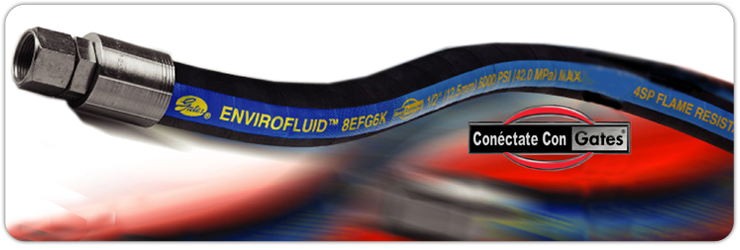# EhisaDistribuidor Autorizado
Usted está en:

## Acero Inoxidable

 o.d (ln.) PARED(IN) i.d (IN) TIPO wt./ft(IBS) 1/8" o.d . 125 0.035 0.055 S/COST 0.034 3/16"O.D . 188 0.035 0.118 S/COST-C/COST 0.057 1/4"O.D . 250 0.035 0.180 S/COST-C/COST 0.080 . 250 0.049 0.152 S/COST-C/COST 0.105 . 250 0.065 0.12 S/COST 0.128 5/16"O.D 0.313 0.035 0.243 S/COST 0.104 0.313 0.049 0.215 S/COST 0.138 0.313 0.065 0.183 S/COST 0.172 3/8"O.D 0.375 0.028 0.319 C/COST 0.100 0.375 0.035 0.305 S/COST-C/COST 0.127 0.375 0.049 0.277 S/COST-C/COST 0.171 0.375 0.065 0.245 S/COST-C/COST 0.215 0.375 0.083 0.209 S/COST 0.259 1/2" O.D . 500 0.035 0.430 S/COST-C/COST 0.174 . 500 0.049 0.402 S/COST-C/COST 0.236 . 500 0.065 0.370 S/COST-C/COST 0.302 . 500 0.083 0.334 S/COST-C/COST 0.370 . 500 0.095 0.310 S/COST-C/COST 0.411 5/8"O.D 0.625 0.035 0.555 S/COST-C/COST 0.221 0.625 0.049 0.527 S/COST-C/COST 0.301 0.625 0.065 0.495 S/COST-C/COST 0.389 0.625 0.083 0.459 S/COST-C/COST 0.481 0.625 0.095 0.435 S/COST-C/COST 0.538 3/4"O.D 0.750 0.035 0.680 S/COST-C/COST 0.267 0.750 0.049 0.652 S/COST-C/COST 0.367 0.750 0.065 0.62 S/COST-C/COST 0.476 0.750 0.083 0.584 S/COST-C/COST 0.591 0.750 0.095 0.56 S/COST-C/COST 0.665 0.750 0.109 0.532 S/COST 0.746 0.750 0.120 0.510 S/COST 0.807 7/8"O.D 0.875 0.049 0.777 S/COST-C/COST 0.432 0.875 0.065 0.745 S/COST-C/COST 0.562 0.875 0.095 0.685 S/COST 0.791 1"O.D 1.000 0.049 0.902 S/COST-C/COST 0.498 1.000 0.065 0.870 S/COST-C/COST 0.649 1.000 0.083 0.834 S/COST-C/COST 0.813 1.000 0.095 0.810 S/COST-C/COST 0.918 1.000 0.109 0.782 S/COST-C/COST 1.037 1.000 0.120 0.760 S/COST-C/COST 1.128 1_1/8"O.D 1.125 0.065 0.995 C/COST 0.736 1_1/4"O.D 1.250 0.065 1.120 S/COST-C/COST 0.823 1.250 0.095 1.060 S/COST-C/COST 1.172 1.250 0.120 1.010 S/COST-C/COST 1.448 1_1/2O.D 1.500 0.065 1.370 S/COST-C/COST 0.996 1.500 0.095 1.310 S/COST-C/COST 1.426 1.500 0.120 1.260 S/COST-C/COST 1.769 1-3/4O.D 1.750 0.065 1.620 C/COST 1.170 2"O.D 2.000 0.065 1.870 C/COST 1.343 2.000 0.095 1.810 C/COST 1.933 2-1/2"O.D 2.500 0.065 2.370 C/COST 1.690